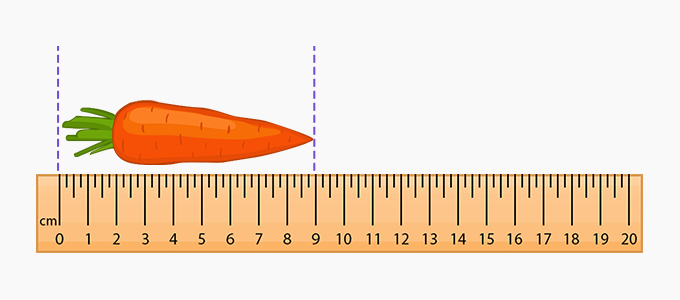# Equal Sign - Definition with Examples

The Complete K-5 Math Learning Program Built for Your Child

• 40 Million Kids

Loved by kids and parent worldwide

• 50,000 Schools

Trusted by teachers across schools

• Comprehensive Curriculum

Aligned to Common Core

## What is Equal Sign?

The equal sign in mathematics describes equality between the values, equations, or expressions written on both sides.

The symbol for equal to is two small horizontal lines placed parallelly.We place the ‘equal to’ sign is between two things that are the same or equal.Examples:

1 + 1 = 2

This means that 1 + 1 is equal to 2

\$1 = 100 cents

This means that 1 dollar is equivalent to 100 cents.

 Fun Facts There are many uses of the equal sign in mathematics. One of the most import use is in mathematical equations. An equation cannot be written without an equal to sign.          4x + 12 = 24

An equation contains variables, constants and an equal to sign in the form of expression. In every equation, the expression on the left-hand side (LHS) is equal to the expression on the right-hand side (RHS).Measurement shows the amount or quantity of something.

Let us see how to use the equal to sign in measurement.

Kelly is given the task of measuring the length of a fork. She uses a centimeter scale to measure the length.She finds the distance between the start and the end point of the fork.

The length of the fork = 18 cm.

(length ‘is equal’ to 18 cm)

Won Numerous Awards & Honors